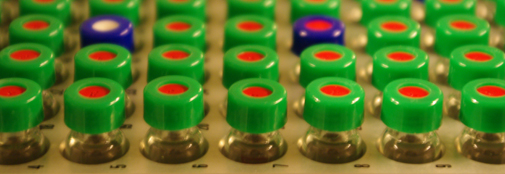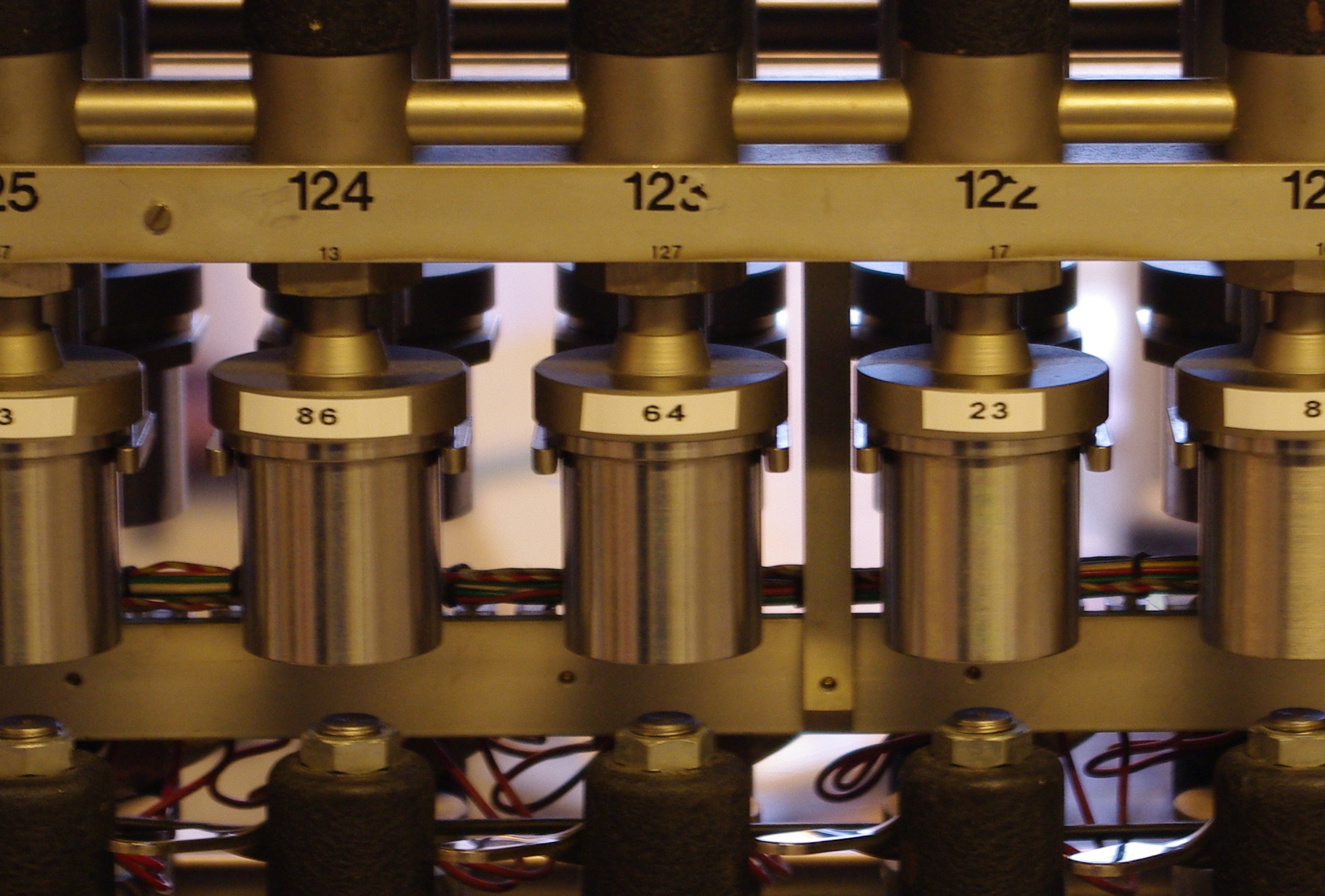#Water isotope measurements at the Centre for Ice and Climate

Measuring the isotopic composition of water is one of the main experimental activities at the Centre for Ice and Climate, and the history of water isotope measurements goes back to Willi Dansgaard's pioneer work from the 1950ies.
Today, the Centre for Ice and Climate uses two different setups for water isotope measurements: One that uses the equilibration technique and one that uses high temperature conversion. Both techniques make use of a mass spectrometer.
In addition, we are involved in testing and development of novel equipment that uses laser absorption methods.

## Equilibration technique

First the ice samples are melted and transferred to small containers. Then the air in the containers is substituted with CO2. The containers are then stirred slightly for a few hours and during this period the CO2 exchanges with the water. Afterwards the isotopic composition of the oxygen in the water (H2O) has equilibrated with the isotopic composition of the oxygen in the CO2. That is to say that the O18/O16 ratio in the water is the same as the O18/O16 ratio in the CO2. The CO2 gas is then extracted from the containers and transferred to a mass spectrometer that has been adjusted to measure the abundance of CO2 molecules with masses 44 (C12O16O16) and 46 (C12O16O18). The mass 46/44 ratio can then be used to calculate O18/O16 ratios for the ice samples.In these metal beakers, the melted ice samples equilibrate with CO2, so that the isotopic composition of the sample and CO2 is the same. The isotopic composition of this CO2 is then measured in a mass spectrometer.

## High temperature conversion

First the ice samples are melted and transferred to small containers. The water is then extracted from the containers and injected into an oven with a temperature of 1400°C. At this temperature, the water molecules react with a carbon column in the oven to form H2 gas and CO gas. The gases are separated and carried by a flow of helium into a mass spectrometer. This mass spectrometer is calibrated to measure masses 2 (H1H1) and 3 (H1H2) first and then proceed to measure masses 28 (C12O16) and 30 (C12O18). The mass 3/2 ratio can then be used to calculate the H2/H1 ratios for the ice samples while the mass 30/28 ratio can be used to calculate O18/O16 ratios for the ice samples.